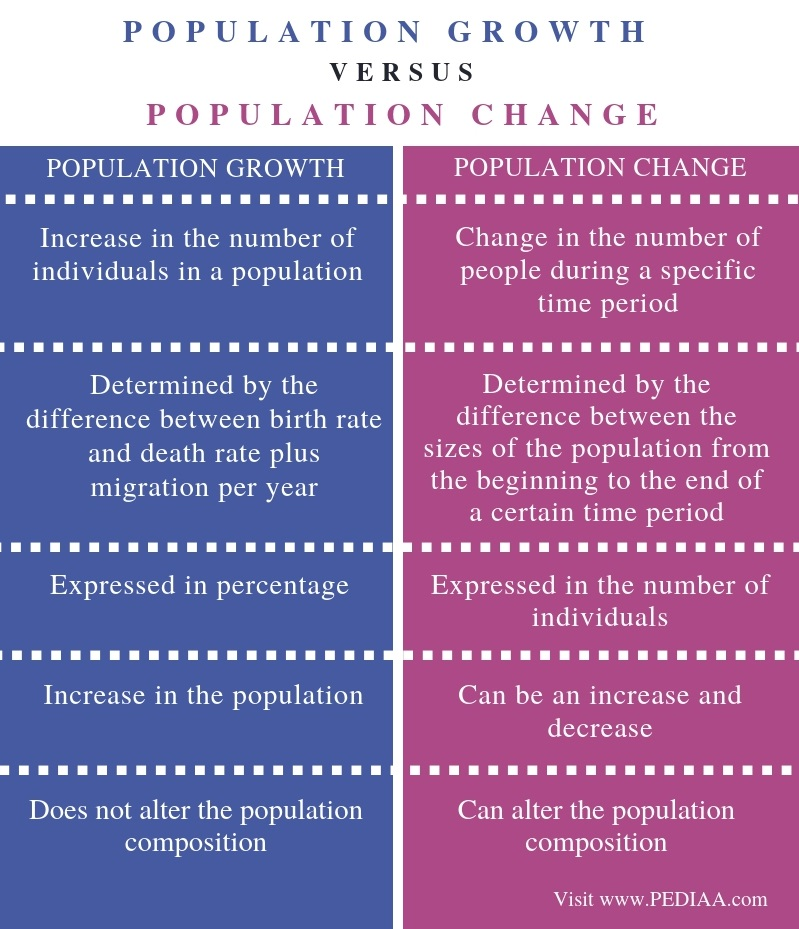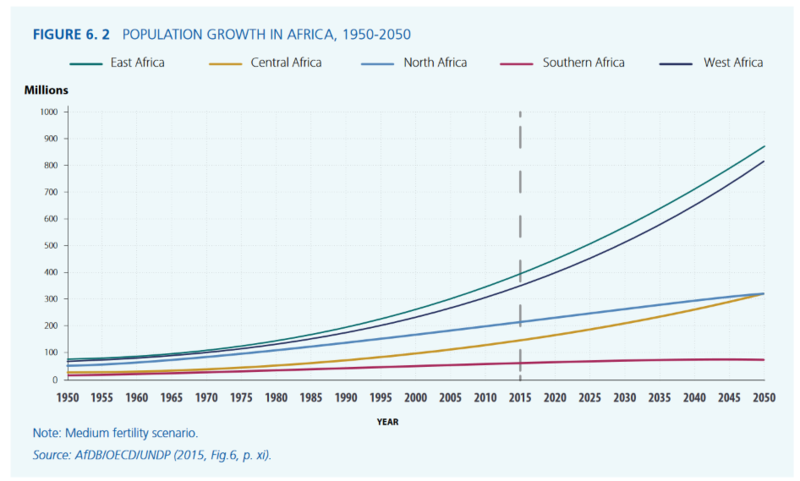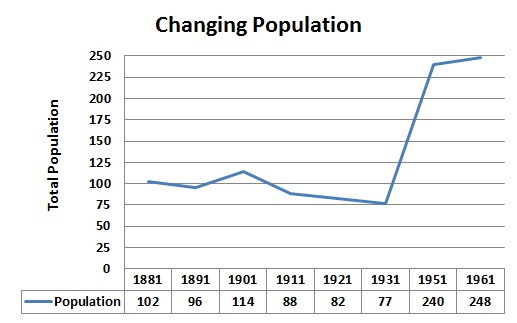# Difference Between Population Growth and Population Change

The main difference between population growth and population change is that the population growth is the increase in population due to natural growth and migration whereas the population change is the change in the population composition. Furthermore, population growth has no influence on the population composition while population change alters the composition of the population.

Population growth and population change are two parameters of ecology used to describe a particular population of living organisms.

### Key Areas Covered

1. What is Population Growth
– Definition, Calculation, Influence
2. What is Population Change
– Definition, Calculation, Influence
3. What are the Similarities Between Population Growth and Population Change
– Outline of Common Features
4. What is the Difference Between Population Growth and Population Change
– Comparison of Key Differences

### Key Terms

Birth Rate, Death Rate, Migration, Population Change, Population Growth## What is Population Growth

Population growth is the increase in the number of individuals in a population. The two factors involved in the increase in the number of individuals in a population are birth rate and migration into the population. Meanwhile, the death rate and the migration out of the population decrease the number of individuals in a population. Therefore, the formula for the population growth is as follows:

Population Growth = (birth rate + immigration) – (death rate + emigration)Figure 1: Population Growth in Africa, 1950 – 2050

Population growth is an exponential process; hence, the population increases by a constant amount over a period of time. It is affected by the number of individuals already present in the population. Other factors such as fertility and rates of motility also affect the growth of the population. This means population growth cannot be considered as a linear process involved in the constant doubling of individuals. Moreover, population growth is one of the main problems behind many ecological problems since it increases the demand for resources.

## What is Population Change

Population change is the difference in the size of the population from the beginning to the end of a certain period of time. Some populations decrease their size while others such as the human population increase in size. Hence, populations are not stable. The change in the population also changes the composition of the population defined by the birth and the death rates, sex ratios, life expectancy as well as the migration rates.Figure 2: Population Change in Albrighton, Shrewsbury

## Similarities Between Population Growth and Population Change

• Population growth and population change are two parameters used to describe the changes which occur in a particular population.
• Both can interpret the increase in the number of population.

## Difference Between Population Growth and Population Change

### Definition

Population growth refers to the increase in the number of individuals in a population while population change refers to the change in the number of people during a specific time period. This explains the basic difference between population growth and population change.

### Determination

Another difference between population growth and population change is in their determination. Population growth can be determined by the difference between birth rate and death rate plus migration per year while population change is determined by the difference between the sizes of the population from the beginning to the end of a certain time period.

### Expression

Also, the population growth is expressed in percentage while population change is expressed in the number of individuals.

### Significance

Furthermore, population growth is the increase in the population while population change can be either an increase or decrease.

### Population Composition

One other difference between population growth and population change is that the population growth does not alter the population composition while population change can alter the population composition.

### Examples

Population growth is 1.1% per year while the human population change is 0.804 billion from 1991 to 2001.

### Conclusion

Population growth is the increase in the number of population while population change is the change in the number of individuals in the population, which can be either increase or decrease. The main difference between population growth and population change is the type of change in the population.

##### Reference:

1. “Population Growth.” Lenntech Water Treatment & Purification, LENNTECH, Available Here
2. “Factors of Population Change.” Population Forecasts | Coffs Harbour City Council, Id – the Population Experts for Coffs Harbour City Council, Available Here

##### Image Courtesy:

1. “Population Growth in Africa, 1950 – 2050” By UNESCO – http://unesdoc.unesco.org/images/0024/002439/243938e.pdf (CC BY-SA 3.0-igo)  via Commons Wikimedia
2. “Population change in Albrighton, Shrewsbury over time” By Anthony Marriott – Excel (CC BY-SA 3.0) via Commons Wikimedia